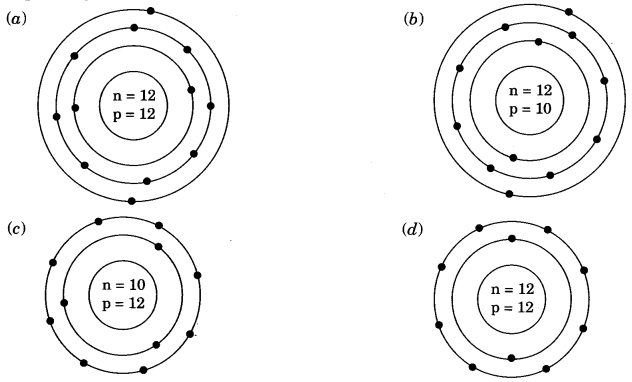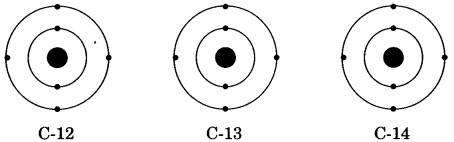# CBSEtips.in

## Saturday, 20 February 2021

### CBSE Class 9 Science - MCQ and Online Tests- Chemistry - Unit 4 - Structure of the Atom

#### CBSE Class 9 Science – MCQ and Online Tests – Chemistry – Unit 4 – Structure of the Atom

Every year CBSE  schools conducts Annual Assessment exams for 6,7,8,9,11th standards. These exams are very competitive to all the students. So our website provides online tests for all the 6,7,8,9,11th standard’s subjects. These tests are also very effective and useful for those who preparing for any competitive exams like Olympiad etc. It can boost their preparation level and confidence level by attempting these chapter wise online tests.

These online tests are based on latest CBSE syllabus. While attempting these our students can identify the weak lessons and continuously practice those lessons for attaining high marks. It also helps to revise the NCERT textbooks thoroughly.

#### CBSE Class 9 Science – MCQ and Online Tests – Chemistry – Unit 4 – Structure of the Atom

Question 1.
The first model of an atom was given by
(a) N. Bohr
(b) E. Goldstein
(c) Rutherford
(d) J.J. Thomson

Answer: (d) J.J. Thomson

Question 2.
Atomic models have been improved over the years. Arrange the following atomic models in the order of their chronological order
(i) Rutherford’s atomic model
(ii) Thomson’s atomic model
(ii) Bohr’s atomic model
(a) (i), (ii) and (iii)
(b) (ii), (iii) and (i)
(c) (ii), (i) and (iii)
(d) (iii), (ii) and (i)

Answer: (c) (ii), (i) and (iii)

Question 3.
The ion of an element has 3 positive charges. Mass number of the atom is 27 and the number of neutrons is 14. What is the number of electrons in the ion?
(a) 13
(b) 10
(c) 14
(d) 16

Question 4.
Identify the Mg2+ ion from the figure where, n and p represent the number of neutrons and protons respectively.Question 5.
An atom with 3 protons and 4 neutrons will have a valency of
(a) 3
(b) 7
(c) 1
(d) 4

Question 6.
Which of the following correctly represents the electronic distribution in the Mg atom?
(a) 3, 8, 1
(b) 2, 8, 2
(c) 1, 8, 3
(d) 8, 2, 2

Answer: (b) 2, 8, 2

Question 7.
Which of the following statement is always correct?
(a) An atom has equal number of electrons and protons.
(b) An atom has equal number of electrons and neutrons.
(c) An atom has equal number of protons and neutrons.
(d) An atom has equal number of electrons, protons and neutrons.

Answer: (a) An atom has equal number of electrons and protons.

Question 8.
The number of electrons in an element X is 15 and the number of neutrons is 16. Which of the following is the correct representation of the element?
(a) \(_{15}^{31}\)X
(b) \(_{16}^{31}\)X
(c) \(_{15}^{16}\)X
(d) \(_{16}^{15}\)X

Question 9.
Which of the following are true for an element?
(i) Atomic number = number of protons + number of electrons
(ii) Mass number = number of protons + number of neutrons
(iii) Atomic mass = number of protons = number of neutrons
(iv) Atomic number = number of protons = number of electrons
(a) (i) and (ii)
(b) (i) and (iii)
(c) (ii) and (iii)
(d) (ii) and (iv)

Answer: (d) (ii) and (iv)

Question 10.
Which of the following statements about Rutherford’s model of atom are correct?
(i) Considered the nucleus as positively charged.
(ii) Established that the a-particles are four times as heavy as a hydrogen atom.
(iii) Can be compared to solar system.
(iv) Was in agreement with Thomson’s model.
(a) (i) and (iii)
(b) (ii) and (iii)
(c) (i) and (iv)
(d) only (i)

Answer: (a) (i) and (iii)

Question 11.
Isobars do not differ in the number of
(a) protons
(b) electrons
(c) neutrons
(d) nucleons

Question 12.
Which of the following isotope is used in the treatment of blood cancer?
(a) P-32
(b) I-131
(c) Co-60
(d) any of these

Question 13.
Hydrogen exists in three isotopic forms, \(_{1}^{1}\)H, \(_{1}^{2}\)H, \(_{1}^{3}\)H known as protium, deuterium and tritium. Why are all the isotopes neutral in nature?
(a) Since neutrons are neutral in nature hence isotopes are electrically neutral.
(b) All the isotopes have one electron and one proton, hence they are neutral.
(c) All the isotopes have one proton and one neutron, hence they are neutral.
(d) Increasing number of protons in the isotopes make them neutral.

Answer: (b) All the isotopes have one electron and one proton, hence they are neutral.

Question 14.
How many electrons are present in M-shell of an element with atomic number 20?
(a) 5
(b) 8
(c) 12
(d) 18

Question 15.
There are two atomic species X and Y, such thatWhich of the following statements is true about X and Y?
(a) X and Y are isobars.
(b) X and Y have different chemical properties.
(c) X and Y have different physical properties.
(d) All of these.

Answer: (c) X and Y have different physical properties.

Question 16.
The ion of an element has 3 positive charges. Mass number of the atom is 27 and the number of neutrons is 14. What is the number of electrons in the ion?
(a) 13
(b) 10
(c) 14
(d) 16

Question 17.
An alpha particle is also known as:
(a) subatomic particle
(b) an unionised helium atom
(c) a neutral particle
(d) a doubly-charged helium ion

Answer: (d) a doubly-charged helium ion

Question 18.
There are three isotopes of carbon which are named as C-12, C-13 and C-14 out of which C-12 is the most abundant isotope. In the given structures of 3 isotopes, what will be the composition of the nucleus?(a) C-12 : 6p + 6n, C-13 : 7p + 6n, C-14 : 8p + 6n
(b) C-12 : 6p + 6n, C-13 : 6p + 7n, C-14 : 6p + 8n
(c) C-12 : 6p + 6n, C-13 : 5p + 8n, C-14 : 7p + 7n
(d) C-12 : 6p + 6n, C-13 : 12p + 1n, C-14 : 5p + 9n

Answer: (b) C-12 : 6p + 6n, C-13 : 6p + 7n, C-14 : 6p + 8n

Question 19.
Rutherford’s ‘alpha (a) particles scattering experiment’ resulted in the discovery of
(a) electron
(b) proton
(c) nucleus in the atom
(d) atomic mass

Answer: (c) nucleus in the atom

Question 20.
The charge on an electron is equal to:
(a) 1.6 × 10-19 C of -ve charge
(b) 2.6 × 10-19 C of -ve charge
(c) 1.6 × 10-22 C of -ve charge
(d) 1.6 × 10-23 C of -ve charge

Answer: (a) 1.6 × 10-19 C of -ve charge

Share: